## 1. Introduction

In mathematics, a polynomial is an expression that contains a sum of powers in one or more variables multiplied by coefficients. A polynomial in one variable,, with constant coefficients is like:. We call each item, e.g.,, a term. If two terms have the same power, we call them like terms.

In this tutorial, we’ll show how to add and multiply two polynomials using a linked list data structure.

## 2. Represent a Polynomial with a Linked List

We can use a linked list to represent a polynomial. In the linked list, each node has two data fields: coefficient and power. Therefore, each node represents a term of a polynomial. For example, we can represent the polynomialwith a linked list: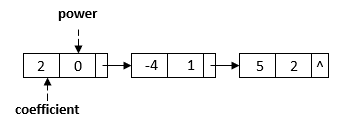We can sort a linked list intime, whereis the total number of the linked list nodes. In this tutorial, we’ll assume the linked list is ordered by the power for the simplicity of our algorithms.

To add two polynomials, we can add the coefficients of like terms and generate a new linked list for the resulting polynomial. For example, we can use two liked lists to represent polynomialsand: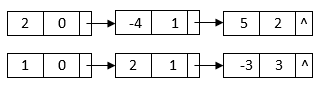When we add them together, we can group the like terms and generate the result: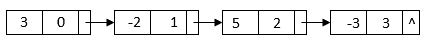Since both linked list are ordered by the power, we can use a two-pointer method to merge the two sorted linked list:In this algorithm, we first create two pointers,and, to the head pointers of the two input polynomials. Then, we generate the new polynomial nodes based on the powers of these two pointers. There are three cases:

1.‘s power is greater than‘s power: In this case, we append a new node with‘s power and coefficient. Also, we moveto the next node.
2.‘s power is greater than‘s power: In this case, we append a new node with‘s power and coefficient. Also, we moveto the next node.
3.andhave the same power: In this case, the new coefficient is the total of‘s coefficient and‘s coefficient. If the new coefficient is not, we append a new node with the same power and the new coefficient. Also, we move bothandto the next nodes.

After that, we continue to append the remaining nodes fromoruntil we finish the calculation on all nodes.

Thefunction can create a new linked list node based on the inputand. Also, it appends the new node to thenode and returns the newnode:This algorithm traverses each linked list node only once. Therefore, the overall running time is, whereandare the number of terms for the two input polynomials.

## 4. Multiply Two Polynomials

To multiply two polynomials, we can first multiply each term of one polynomial to the other polynomial. Suppose the two polynomials haveandterms. This process will create a polynomial withterms.

For example, after we multiplyand, we can get a linked list: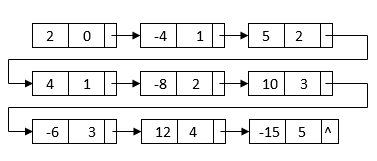This linked list contains all terms we need to generate the final result. However, it is not sorted by the powers. Also, it contains duplicate nodes with like terms. To generate the final linked list, we can first merge sort the linked list based on each node’s power: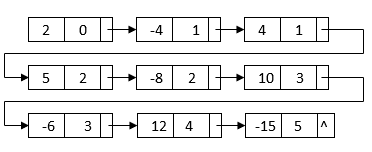After the sorting, the like term nodes are grouped together. Then, we can merge each group of like terms and get the final multiplication result: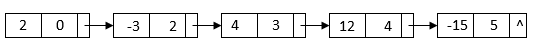We can describe these steps with an algorithm:In this algorithm, we first use a nestedloop to multiply all term pairs from the two polynomials. This takestime, whereandare the number of terms for the two input polynomials. Also, this process creates a linked list withnodes.

Then, we merge sort the linked list based on each node’s power. This process takestime.

After we sort the linked list, we can use a two-pointer approach to merge the like terms:In this approach, we use one pointer as the start of a like term group and use another pointer to traverse the like terms in the same group. Each time we find a like term, we add its coefficient to the start like term node. Once we finish one like term group, we move the start pointer to the next group and repeat the same process to merge like terms. The running time of this process isas we need to visitnodes.

Overall, the running time is.

## 5. Conclusion

In this tutorial, we showed how to represent a polynomial with the linked list data structure. Also, we showed two algorithms to add and multiply two linked list representations of polynomials.